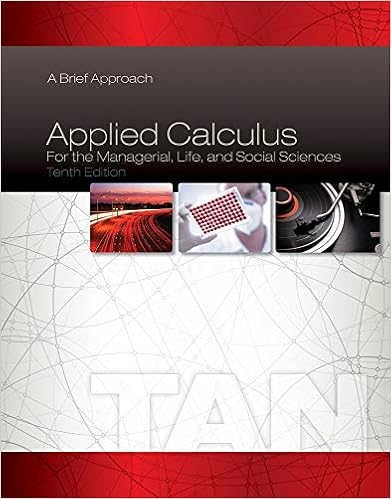# Given the following data what is the gross profit if

• Test Prep
• Ashish1
• 19
• 74% (19) 14 out of 19 people found this document helpful

This preview shows page 8 - 11 out of 19 pages.

##### We have textbook solutions for you!
The document you are viewing contains questions related to this textbook.The document you are viewing contains questions related to this textbook.
Chapter 2 / Exercise 27
Applied Calculus for the Managerial, Life, and Social Sciences: A Brief Approach
TanExpert Verified
59.Given the following data, what is the gross profit if cost of goods sold is determined using the FIFO method?Sales revenue200units at\$20per unitBeginning inventory60units at\$12per unitPurchases210units at\$13per unita)\$1,460b)\$2,540c)\$1,400d)cannot be determined because the number of units in ending inventory is not given
60.Given the following data, what is the gross profit if cost of goods sold is determined using the LIFO method?Sales revenue200units at\$20per unitBeginning inventory60units at\$12per unitPurchases210units at\$13per unit
61.Given the following data, what is the gross profit if cost of goods sold is determined using the average cost method?
##### We have textbook solutions for you!
The document you are viewing contains questions related to this textbook.The document you are viewing contains questions related to this textbook.
Chapter 2 / Exercise 27
Applied Calculus for the Managerial, Life, and Social Sciences: A Brief Approach
TanExpert Verified
CHAPTER 6Accounting                                                 Aamer Waheed Satti
Table 5January 1 inventory balance90 units at \$10 per unitMarch 2 purchase50 units at \$11 per unitJuly 8 purchase80 units at \$10 per unitNovember 15 purchase30 units at \$12 per unitDecember 31 inventory balance 75 units62.Referring to Table 5, the cost of ending inventory using the periodic LIFO method would be:
63.Referring to Table 5, cost of goods sold calculated under the periodic FIFO method would be:a)\$1,800b)\$2,160c)\$1,910d)\$1,850
64.Referring to Table 5, assuming all goods are sold throughout the year for \$17 per unit, grossprofit calculated under the periodic FIFO method would be:
65.Referring to Table 5, assuming all goods are sold throughout the year for \$19 per unit, grossprofit calculated under the periodic LIFO method would be:
66.Which statement addresses the consistency principle?
CHAPTER 6Accounting                                                 Aamer Waheed Satti
•••